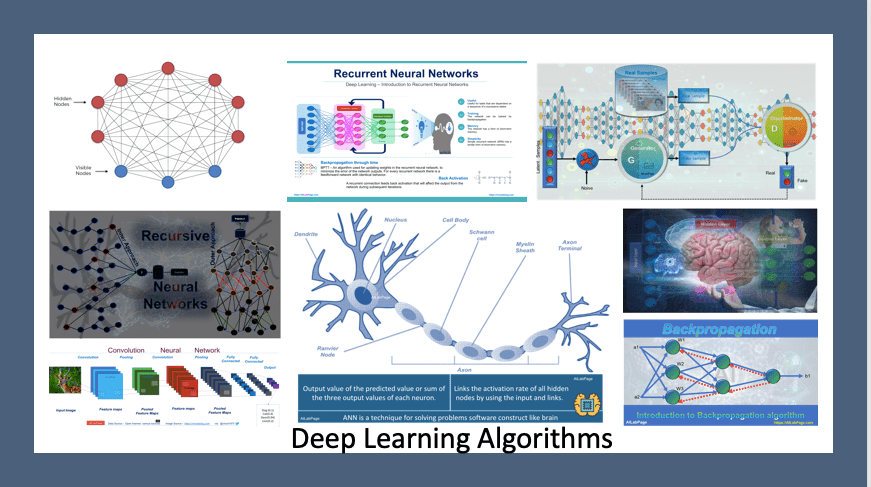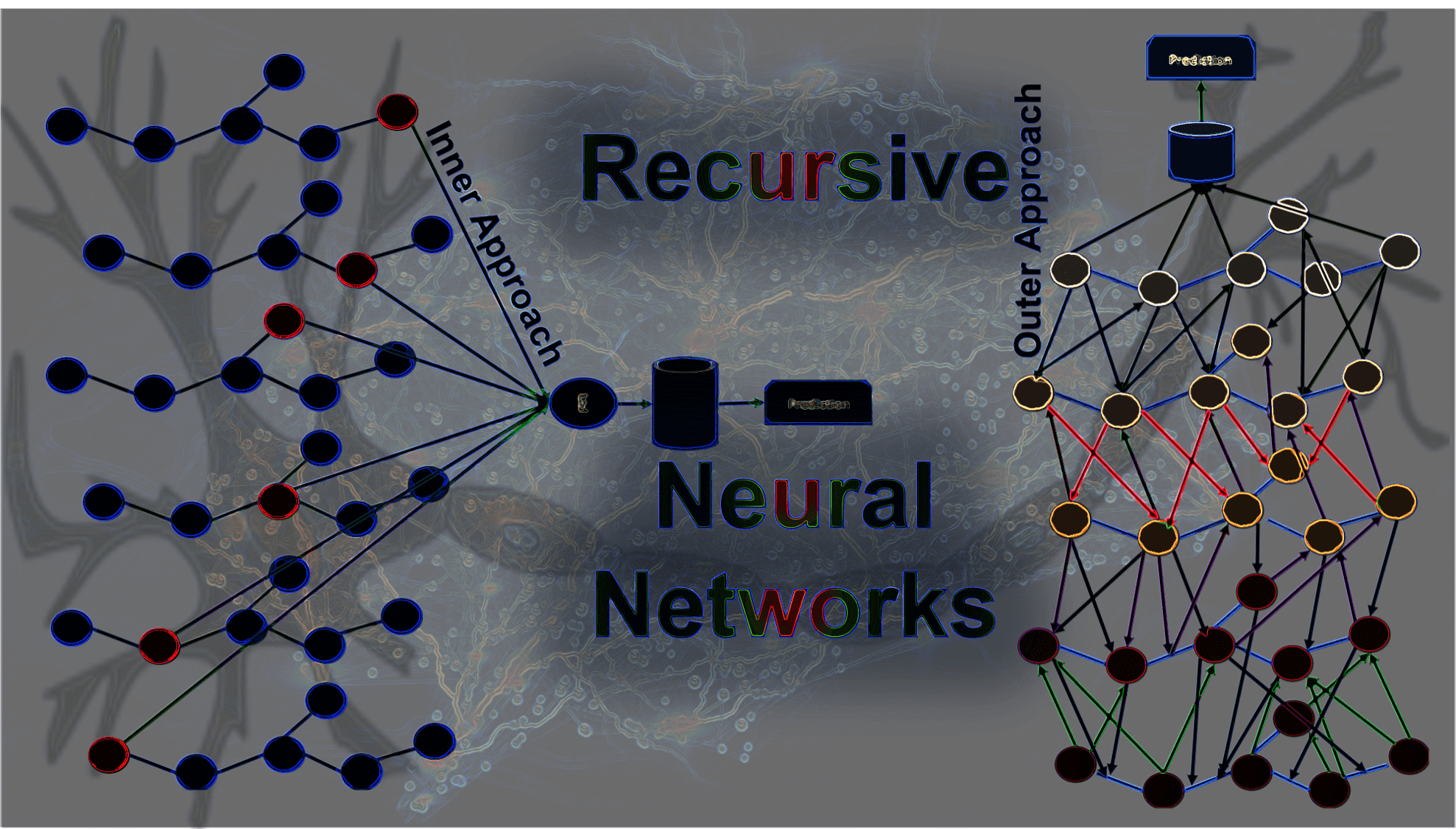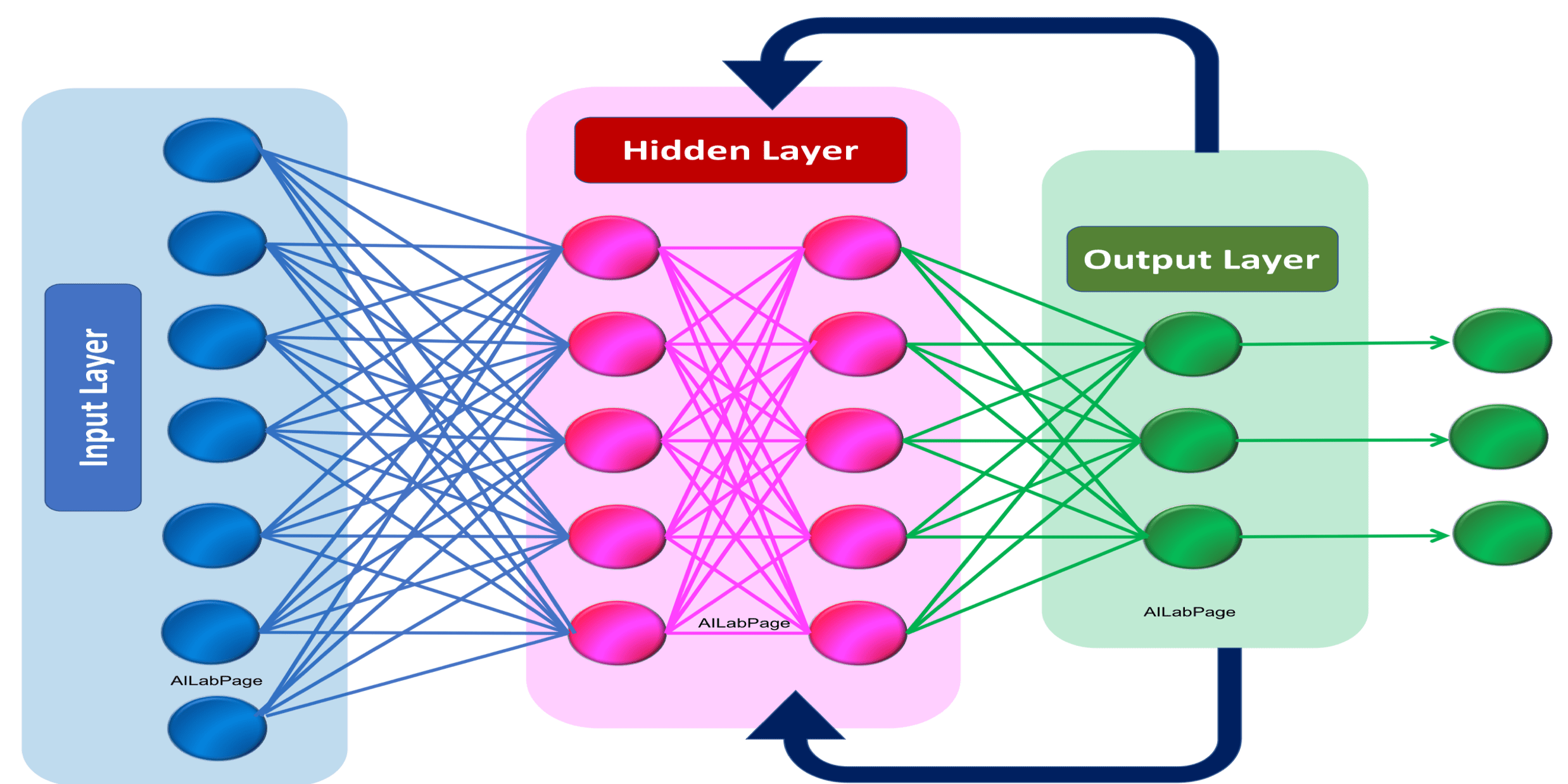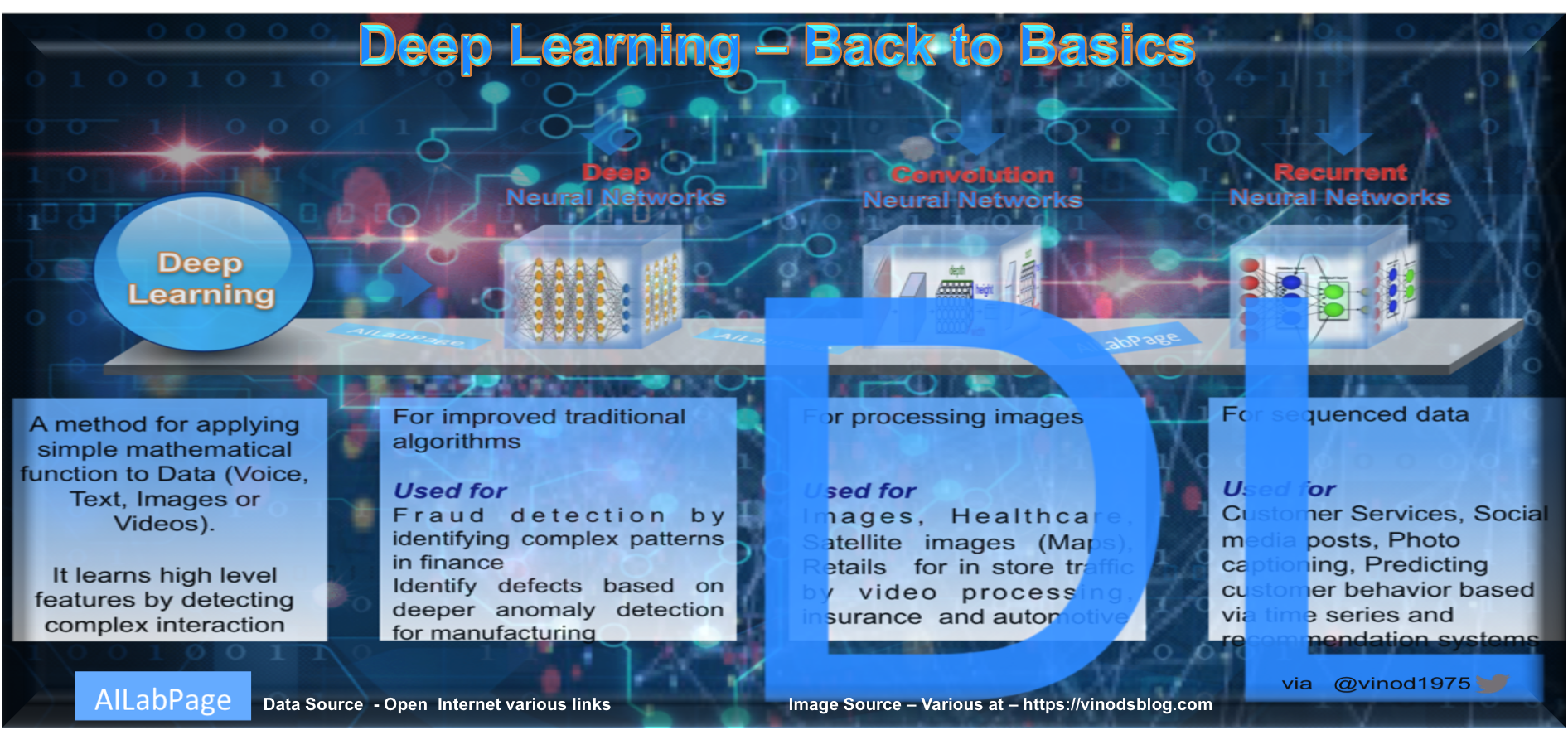# Category: Algorithm### Deep Learning Algorithms — The Basic Guide

Deep learning leverages autonomous learning mechanisms that depend on simulated neural networks, commonly referred to as artificial neural networks (ANNs), to replicate the intricate cognitive operations of the brain implicated in information processing. During the process of training, algorithms endeavor to ascertain significant attributes, organize entities, and unveil consequential patterns within the data via the utilization of latent components in the input distribution.### Deep Learning – Introduction to Recursive Neural Networks

Recursive neural networks belong to the same family of models as deep neural networks, given that they can be seen as a modification of them. Usually, these results are produced by systematically applying a consistent set of weights to the arranged inputs in a repetitive manner. This occurrence happens consistently across all points due to identical causative elements. Recurrent Neural Networks (RNNS) are a group of structured designs that operate on directed acyclic graphs, tailored to handle organized inputs.### Deep Learning – Deep Convolutional Generative Adversarial Networks Basics

Generative Adversarial Networks are a class of algorithms used in the unsupervised learning environment. As the name suggests they are called Adversarial Networks because they are made up of two competing neural networks. Both networks compete with each other to achieve a zero-sum game..### Deep Learning – Introduction to Recurrent Neural Networks

“Artificial neural networks (ANNs) are biologically inspired computing code with number of simple, highly interconnected processing elements for simulating human brain workings to process information model”.### Naive Bayes Algorithm – The basics you need to know

The naive Bayes algorithm is a method set of probabilities. For each attribute from each class set, it uses probability to make predictions. This algorithm falls under a supervised machine-learning approach. The data model that comes out of this effort is called as “Predictive Model” with probabilistic problems at the foundation level### Deep Learning – Mandate for Humans, Not Just Machines

Deep Learning terminology can be quite overwhelming to newcomers.. This blog post covers important aspect of deep learning which can be defined as set of techniques that uses neural networks to simulate human decision-making skills. #AILabPage